# Multiplying by a 1 digit number

##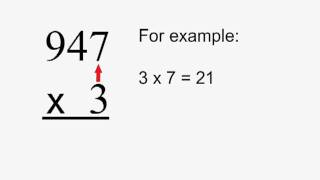By MathNook

MathNook presents Multiplying by a 1 digit number an educational video resource on math.# Multiplying 4 digits by 1 digit with visual models

##By Khan Academy

It's helpful sometimes to use a grid when multiplying multi-digit numbers. Here's an example using a 4 digit number times a 1 digit number.# Multiplying: 3 digits times 1 digit | Multiplication and division | Arithmetic | Khan Academy

##By Khan Academy

How about this example? We're going to multiply a 3 digit number by a 1 digit number. Again, no carrying involved.# Multiplying 1-digit numbers

##By Khan Academy

Use arrays to show different ways to multiply and get the same solution.# Multiplying Single Digit Numbers - Arrays & the Commutative Property (3.OA.1, 3.OA.3)

##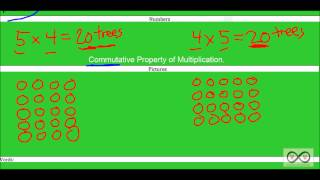By Worksheets and Walkthroughs

This video walkthrough guides the students through two single digit multiplication word problems. Solutions are represented as arrays while proving the commutative property.# Multiplying: 2 digits times 1 digit (with carrying) | Arithmetic | Khan Academy

##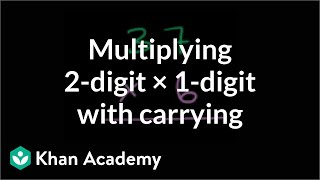By Khan Academy

We use something called the standard method which involves writing the larger of the two numbers on top. Watch.# Multiplying: 4 digits times 1 digit (with carrying) | Arithmetic | Khan Academy

##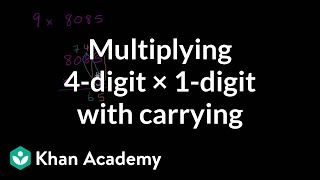By Khan Academy

This is getting fun! We're now multiplying FOUR digits times one digit. Remember to carry!# Multiplying: 3 digits times 1 digit (with carrying) | Arithmetic | Khan Academy

##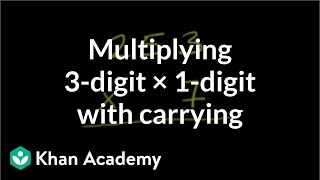By Khan Academy

We're carrying numbers in this example. Everyone needs a hand now and then, right?# FOIL method for multiplying binomials example 2

##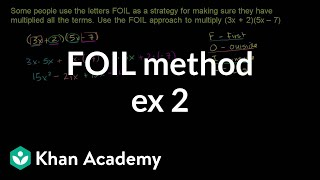By Khan Academy

FOIL method for multiplying binomials example 2# Complex Numbers - Multiplying and Dividing

##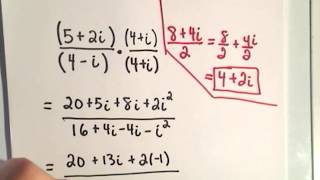By PatrickJMT

This video lesson contains a few example problems on multiplying and dividing complex numbers. For multiplication, the FOIL method is discussed, and for division, the idea of complex conjugates is discussed.# Multiplying binomials and polynomials | Algebra Basics | Khan Academy

##By Khan Academy

This instructor in this ten-minute video, Sal Khan, demonstrates how to multiply polynomials. Mr. Khan uses the Paint Program (with different colors) to illustrate his points. Sal Khan is the recipient of the 2009 Microsoft Tech Award in Education. The student or educator may want to open the video to 'full screen' as the instructor is using a black background and the writing is small# Multiplying Polynomials

##By Dr.Steeve Warner

Multiplying Polynomials# Multiplying polynomials and binomials

##By MathPlanetVideos

Expand the expression (3x+4)(x2−2)# Multiplying and dividing with integers

##By MathPlanetVideos

alculate the following expressions (−4)⋅(−12),−123# Multiplying Polynomials - Introduction

##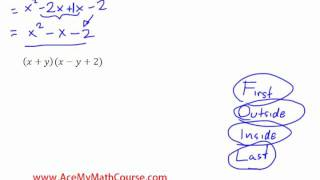By AcademicLeadersEd

Multiplying Polynomials - Introduction an educational video resources on math.# Multiplying Polynomials Animation.mov

##By Alice Keeler

Multiplying Polynomials Animation.mov# Example 3: Multiplying a monomial by a polynomial | Algebra I | Khan Academy

##By Khan Academy

Example 3: Multiplying a monomial by a polynomial | Algebra I | Khan Academy# Example 1: Multiplying binomials to get a difference of squares | Algebra I | Khan Academy

##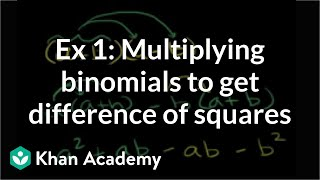By Khan Academy

Example 1: Multiplying binomials to get a difference of squares | Algebra I | Khan Academy# Example 2: Multiplying a binomial by a polynomial | Algebra I | Khan Academy

##By Khan Academy

Example 2: Multiplying a binomial by a polynomial | Algebra I | Khan Academy# Example 1: Multiplying two monomials with a single variable | Algebra I | Khan Academy

##By Khan Academy

This video from the Khan Academy offers explanation on how to multiply with polynomials. Sample problems are offered and a step-by-step walkthrough for each problem is offered as well.